# How to use the diluting solution

What you need:
・Bottled ink
・Diluting solution
・Dropper
・Measuring cup
・Stirrer
・Empty ink bottle (if storing your new ink)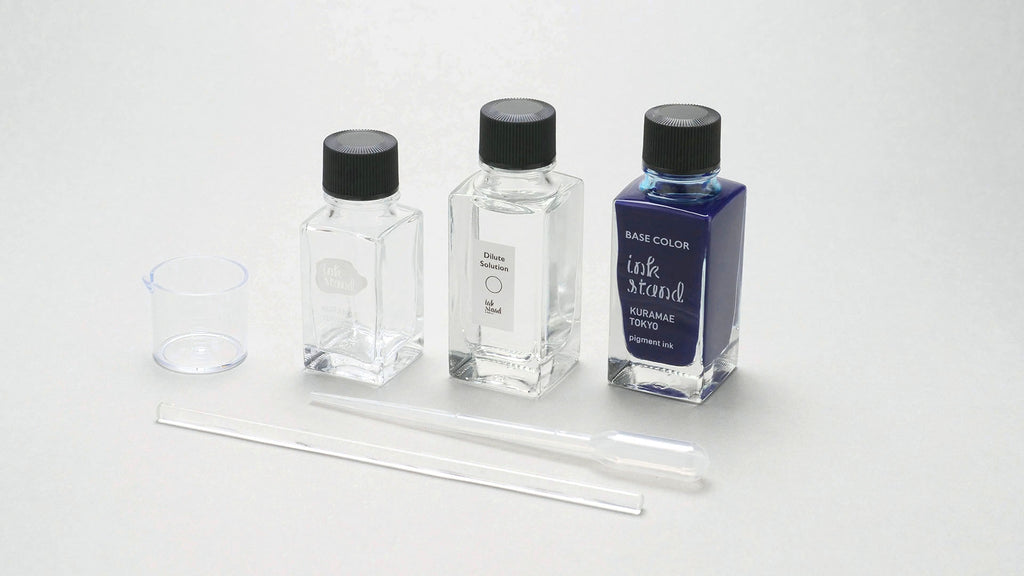### For a single application

Using the dropper, add the required amount of ink and diluting solution to the measuring cup.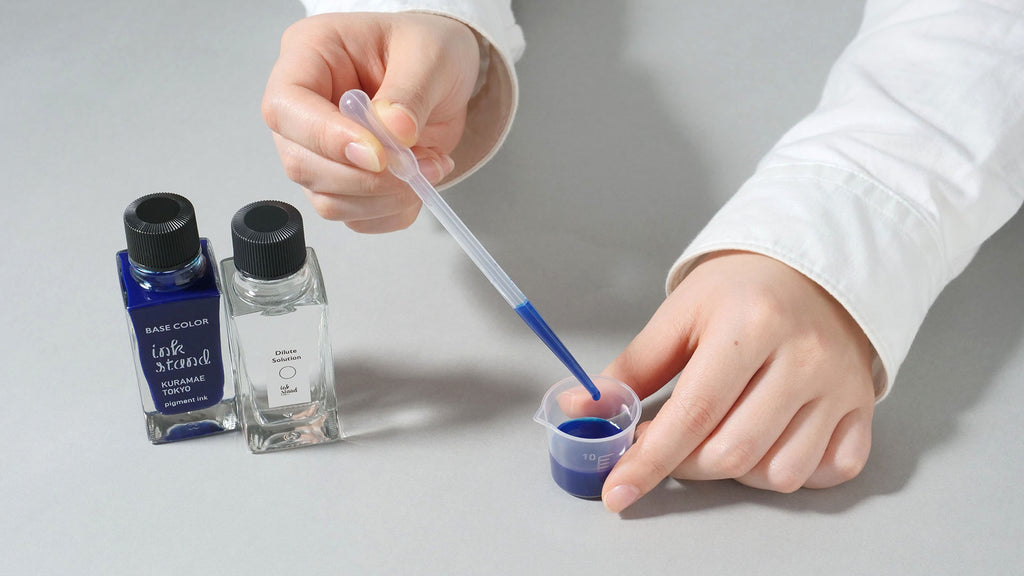Mix well using a stirrer and test the colour on paper. Repeat the process until the desired colour is achieved.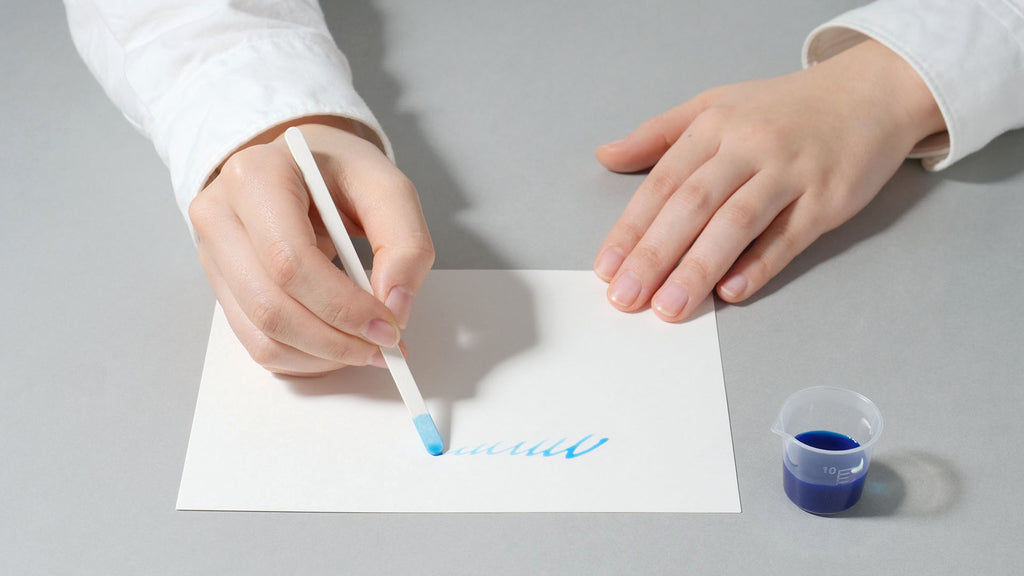To keep a record of the dilution ratio, note the number of drops of ink to diluting solution used.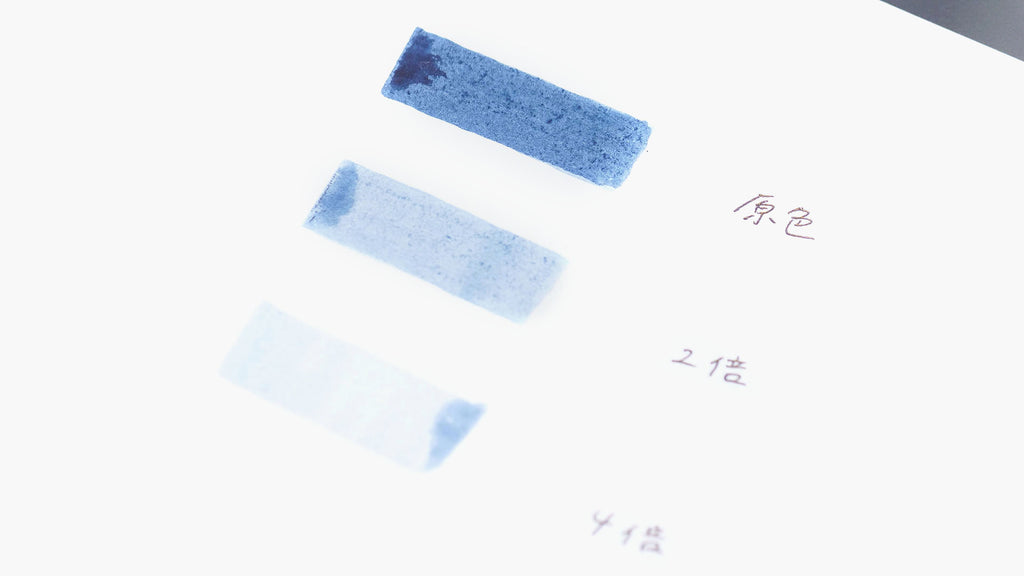### To make a bottle of ink

Step 1: Mix the colour
Using the dropper, add drops of ink and diluting solution to the measuring cup, recording the number of drops of each.
Mix well using a stirrer and test the colour on paper. Repeat the process until the desired colour is achieved.

Step 2: Convert the quantity
Once the colour is finalised, use the following formula to calculate the amount of ink and diluting solution required to make a bottle.
Example:
・Your colour contains 2 drops of ink to 5 drops of diluting solutio
・You would like to make 22ml of ink (total volume)

① A = total volume ÷ (number of drops of ink + number of drops of diluting solution)
Eg. A = 22ml ÷ (2 + 5) = 3.1

② Amount of ink required = A x number of drops of ink Amount of diluting solution required = A x number of drops of diluting solution
Eg.
Amount of ink required = 3.1 x 2 = 6.2ml
Amount of diluting solution required = 3.1 x 5 = 15.5ml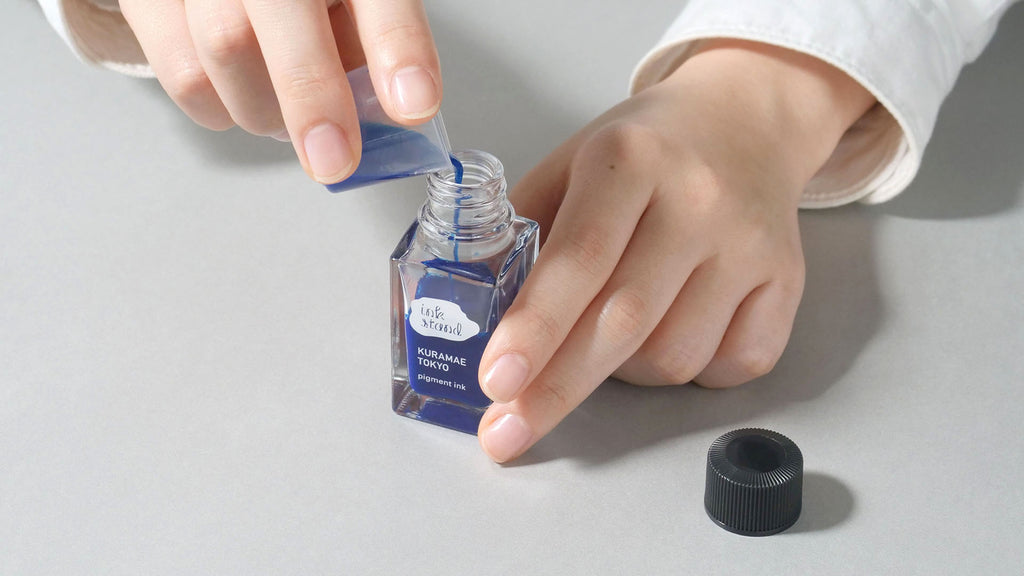Step 3: Make the ink
Using the measuring cup (with 1ml increments), measure and add the calculated amounts of ink and diluting solution to the empty ink bottle. Screw the cap and shake well to finish.